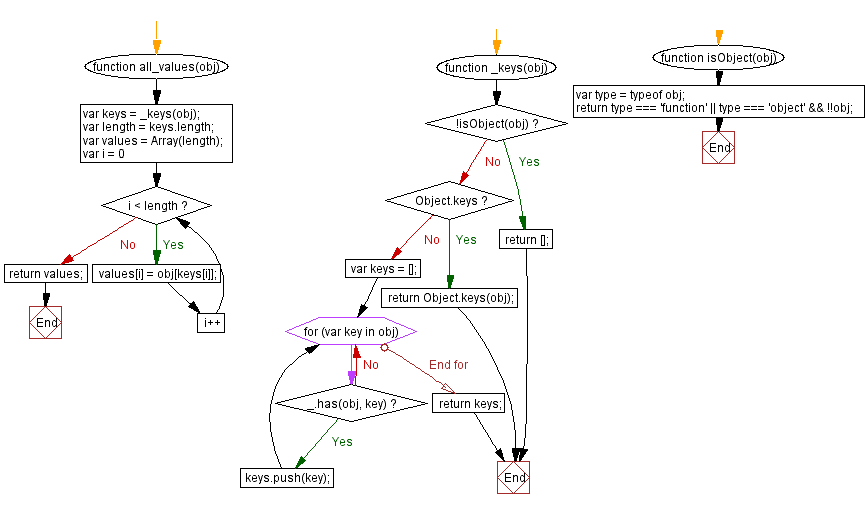# JavaScript: Retrieve all the values of an object's properties

## JavaScript Object: Exercise-14 with Solution

Write a JavaScript function to retrieve all the values of an object's properties.

Sample Solution: -

HTML Code:

``````<!DOCTYPE html>
<html>
<meta charset="utf-8">
<title>JavaScript function to retrieve all the values of an object's properties.</title>
<body>

</body>
</html>
```
```

JavaScript Code:

``````function all_values(obj) {
var keys = _keys(obj);
var length = keys.length;
var values = Array(length);
for (var i = 0; i < length; i++) {
values[i] = obj[keys[i]];
}
return values;
}
function _keys(obj)
{
if (!isObject(obj)) return [];
if (Object.keys) return Object.keys(obj);
var keys = [];
for (var key in obj) if (_.has(obj, key)) keys.push(key);
return keys;
}
function isObject(obj)
{
var type = typeof obj;
return type === 'function' || type === 'object' && !!obj;
}
console.log(all_values({red: "#FF0000", green: "#00FF00", white: "#FFFFFF"}));
```
```

Sample Output:

```["#FF0000","#00FF00","#FFFFFF"]
```

Flowchart:Live Demo:

See the Pen javascript-object-exercise-14 by w3resource (@w3resource) on CodePen.

Improve this sample solution and post your code through Disqus

What is the difficulty level of this exercise?

﻿

## JavaScript: Tips of the Day

JavaScript: eval() function

```const sum = eval('10*10+5');
```

eval evaluates codes that's passed as a string. If it's an expression, like in this case, it evaluates the expression. The expression is 10 * 10 + 5. This returns the number 105.

Ref: https://bit.ly/323Y0P6